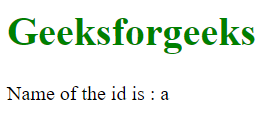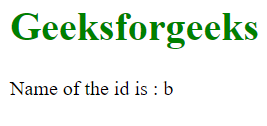GFG App
Open AppBrowser
Continue

# How to print object by id in an array of objects in JavaScript ?

We have an array of objects and in every object, there is a key named id, whose value is a number.

There are many approaches to solving this problem which are following

Using Array.filter( )  method is used for creating a new array from an existing array after applying some conditions.

Example:

## HTML

 `<``h1` `style``=``"color:green"``> ` `    ``Geeksforgeeks ` ` ` `<``p``>Name of the id is : ` `    ``<``span` `id``=``"geeks"``> ` ` ` ` `  `<``script``> ` `    ``// This is our array of Objects ` `    ``var data = [ ` `        ``{ id: 1, name: "a" }, ` `        ``{ id: 2, name: "b" }, ` `        ``{ id: 3, name: "c" }, ` `        ``{ id: 4, name: "d" }, ` `        ``{ id: 5, name: "e" }, ` `        ``{ id: 6, name: "f" }, ` `    ``]; ` `     `  `    ``let idYouWant = 1; ` `    ``let propertyYouWant = "name"; ` `     `  `    ``// Using Array.filter( ) method ` `    ``// we are iterating through each ` `    ``// items in the array and ` `    ``// checking which item's ` `    ``// id value is equal to the id we want ` `     `  `    ``let res = data.filter((item) => { ` `        ``return item.id == idYouWant; ` `    ``}); ` `     `  `    ``// After using filter method we got an array ` `    ``// of object. Now take its first element and ` `    ``// use its 'propertyYouWant' key ` `    ``let exactRes = res[propertyYouWant]; ` `     `  `    ``// Printing the property we want ` `    ``document.getElementById("geeks").innerText = exactRes; ` ``

Output:Print object by id in an array of objects

Using Array.find( ): Using Array.find( ) first we are searching in which object the given id exists, then we extract the name property from that object.

Example:

## HTML

 `<``h1` `style``=``"color:green"``>Geeksforgeeks ` `<``p``>Name of the id is : ` `    ``<``span` `id``=``"geeks"``> ` ` ` ` `  `<``script``> ` `    ``// This is our array of Objects ` `    ``var data = [ ` `        ``{ id: 1, name: "a" }, ` `        ``{ id: 2, name: "b" }, ` `        ``{ id: 3, name: "c" }, ` `        ``{ id: 4, name: "d" }, ` `        ``{ id: 5, name: "e" }, ` `        ``{ id: 6, name: "f" }, ` `    ``]; ` `     `  `    ``let idYouWant = 2; ` `    ``let propertyYouWant = "name"; ` `     `  `    ``// Using Array.find( ) we are searching ` `    ``// in which object our searching id present ` `     `  `    ``let res = data.find((item) => { ` `        ``return item.id == idYouWant; ` `    ``}); ` `     `  `    ``// Now print the property which you want from ` `    ``// the object res console.log(res[propertyYouWant]) ` `    ``document.getElementById("geeks").innerText =  ` `            ``res[propertyYouWant]; ` ``

Output:Print object by id in an array of objects

Using for loop: Using for loop first we are iterating the array and search in which object the given id is present and after that, we are printing the property we wanted.

Example:

## HTML

 `<``h1` `style``=``"color:green"``>Geeksforgeeks ` `<``p``>Name of the id is : ` `    ``<``span` `id``=``"geeks"``> ` ` ` ` `  `<``script``> ` `    ``// This is our array of objects ` `    ``var data = [ ` `        ``{ id: 1, name: "a" }, ` `        ``{ id: 2, name: "b" }, ` `        ``{ id: 3, name: "c" }, ` `        ``{ id: 4, name: "d" }, ` `        ``{ id: 5, name: "e" }, ` `        ``{ id: 6, name: "f" }, ` `    ``]; ` `     `  `    ``let idYouWant = 2; ` `    ``let propertyYouWant = "name"; ` `     `  `    ``// Iterating over the array using for loop and ` `    ``// searching in which object the id present ` `    ``// After getting the object we print the ` `    ``// property we wanted from the object ` `     `  `    ``for (var i = 0; i < ``data.length``; i++) { ` `        ``if (data[i].id == idYouWant) { ` `            ``// console.log(data[i][propertyYouWant]) ` `            ``document.getElementById("geeks")``.innerText` `=  ` `                    ``data``[i][propertyYouWant]; ` `        ``} ` `    ``} ` ``

Output:Print object by id in an array of objects

My Personal Notes arrow_drop_up